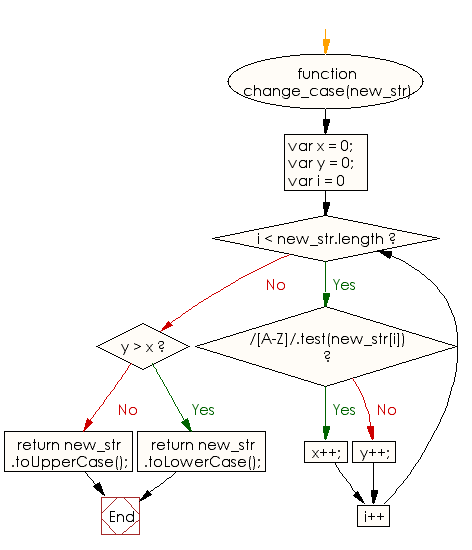# JavaScript: Switch case of the minimum possible number of letters to make a given string written in the upper case or in the lower case

## JavaScript Basic: Exercise-98 with Solution

Write a JavaScript program to switch case of the minimum possible number of letters to make a given string written in the upper case or in the lower case.

Fox example "Write" will be write and "PHp" will be "PHP"

Sample Solution:

HTML Code:

``````<!DOCTYPE html>
<html>
<meta charset="utf-8">
<meta name="viewport" content="width=device-width">
<title> Switch case of the minimum possible number of letters to make a given string written in the upper case or in the lower case</title>
<body>

</body>
</html>
```
```

JavaScript Code:

``````function change_case(new_str) {
var x = 0;
var y = 0;

for (var i = 0; i < new_str.length; i++) {
if (/[A-Z]/.test(new_str[i])) {
x++;
} else y++;
}

if (y > x) return new_str.toLowerCase();
return new_str.toUpperCase();
}

console.log(change_case("Write"))
console.log(change_case("PHp"))
```
```

Sample Output:

```write
PHP
```

Flowchart:ES6 Version:

``````function change_case(new_str) {
let x = 0;
let y = 0;

for (let i = 0; i < new_str.length; i++) {
if (/[A-Z]/.test(new_str[i])) {
x++;
} else y++;
}

if (y > x) return new_str.toLowerCase();
return new_str.toUpperCase();
}

console.log(change_case("Write"))
console.log(change_case("PHp"))
``````

Live Demo:

See the Pen javascript-basic-exercise-98 by w3resource (@w3resource) on CodePen.

What is the difficulty level of this exercise?

Test your Programming skills with w3resource's quiz.

﻿

## JavaScript: Tips of the Day

Returns the sum of the powers of all the numbers from start to end (both inclusive)

Example:

```const sumPower = (end, power = 2, start = 1) =>
Array(end + 1 - start)
.fill(0)
.map((x, i) => (i + start) ** power)
.reduce((a, b) => a + b, 0);
console.log(sumPower(10)); // 385
console.log(sumPower(10, 3)); // 3025
console.log(sumPower(10, 3, 5)); // 2925
```

Output:

```385
3025
2925
```